Download Presentation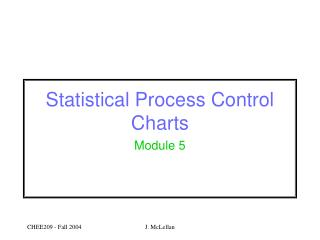Statistical Process Control Charts

# Statistical Process Control Charts - PowerPoint PPT Presentation

Statistical Process Control Charts. Module 5. Goal. monitor behaviour of process using measurements in order to determine whether process operation is statistically stable stable properties not changing in time mean variance on target?. Approach.I am the owner, or an agent authorized to act on behalf of the owner, of the copyrighted work described.
Download Presentation## Statistical Process Control Charts

An Image/Link below is provided (as is) to download presentation

Download Policy: Content on the Website is provided to you AS IS for your information and personal use and may not be sold / licensed / shared on other websites without getting consent from its author.While downloading, if for some reason you are not able to download a presentation, the publisher may have deleted the file from their server.

- - - - - - - - - - - - - - - - - - - - - - - - - - E N D - - - - - - - - - - - - - - - - - - - - - - - - - -
Presentation Transcript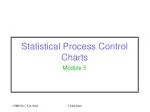### Statistical Process Control Charts

Module 5

J. McLellan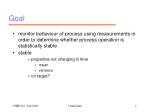Goal
• monitor behaviour of process using measurements in order to determine whether process operation is statistically stable
• stable
• properties not changing in time
• mean
• variance
• on target?

J. McLellan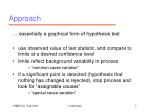Approach

… essentially a graphical form of hypothesis test

• use observed value of test statistic, and compare to limits at a desired confidence level
• limits reflect background variability in process
• “common cause variation”
• if a significant point is detected (hypothesis that nothing has changed is rejected), stop process and look for “assignable causes”
• “special cause variation”

J. McLellan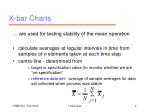X-bar Charts

… are used for testing stability of the mean operation

• calculate averages at regular intervals in time from samples of n elements taken at each time step
• centre-line - determined from
• target or specification value (to monitor whether we are “on-specification”
• reference data set - average of sample averages for data set collected when process was stable

N

1

=

å

X

X

j

N

=

j

1

J. McLellan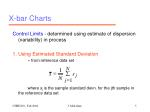X-bar Charts

Control Limits - determined using estimate of dispersion (variability) in process

1. Using Estimated Standard Deviation

• from reference data set

where sjis the sample standard devn. for the jth sample in the reference data set

N

1

=

å

s

s

j

N

=

j

1

J. McLellan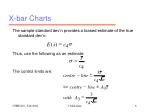X-bar Charts

The sample standard dev’n provides a biased estimate of the true standard dev’n:

Thus, use the following as an estimate:

The control limits are:

=

s

E

(

s

)

c

4

s

s

=

c

4

3

s

-

±

centre

line

c

n

4

Û

-

±

centre

line

A

s

3

3

=

with

A

3

c

n

4

J. McLellan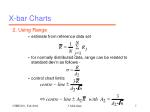X-bar Charts

2. Using Range

• estimate from reference data set
• for normally distributed data, range can be related to standard dev’n as follows -
• control chart limits

N

1

=

å

R

R

j

N

=

j

1

R

s

=

d

2

3

R

-

±

centre

line

d

n

2

3

Û

-

±

=

centre

line

A

R

with

A

2

2

d

n

2

J. McLellan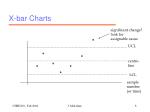X-bar Charts

significant change?

look for

assignable cause

*

UCL

*

*

centre-

line

*

*

*

*

LCL

sample

number

(or time)

J. McLellan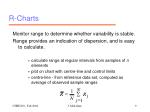R-Charts

Monitor range to determine whether variability is stable.

Range provides an indication of dispersion, and is easy to calculate.

• calculate range at regular intervals from samples of n elements
• plot on chart with centre-line and control limits
• centre-line - from reference data set, computed as average of observed sample ranges

N

1

=

å

R

R

j

N

=

j

1

J. McLellan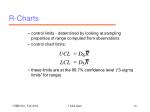R-Charts
• control limits - determined by looking at sampling properties of range computed from observations
• control chart limits:
• these limits are at the 99.7% confidence level (“3-sigma limits” for range)

=

UCL

D

R

4

=

LCL

D

R

3

J. McLellans-Charts

Monitor standard deviation to determine whether variability is stable - standard dev’n provides an indication of dispersion.

• calculate “s” at regular intervals from samples of n elements
• plot on chart with centre-line and control limits
• centre-line - from reference data set, computed as average of observed sample values:

N

1

=

å

s

s

j

N

=

j

1

J. McLellans-Charts
• control chart limits - computed by considering sampling distribution for sample standard dev’n “s”
• chart limits at 99.73% confidence level are -
• define

=

s

E

{

s

}

c

4

2

2

=

-

s

Var

(

s

)

(

1

c

)

4

æ

ö

2

-

1

c

ç

4

÷

±

s

3

s

ç

÷

c

è

ø

4

2

-

3

1

c

4

=

-

B

1

3

c

4

2

-

3

1

c

4

=

+

B

1

4

c

4

J. McLellans-Charts
• chart limits are

B

s

3

B

s

4

J. McLellanMoving Range Charts

How can we measure dispersion when we collect only one data point per sample?

Answer - using the moving range - difference between adjacent sample values:

Use this approach to -

• obtain measure of dispersion for x-bar chart limits
• monitor consistency of variation in the process - MR-chart

=

-

MR

X

X

-

j

j

1

J. McLellanUsing Moving Ranges for X-bar Limits

Calculate average moving range from reference data set:

Convert AMR into an estimate for the standard deviation using the constant “d2” for n=2 sample points:

centre-line - use either a target value, or the average of the samples in the reference data set

N

1

=

=

-

å

AMR

M

R

X

X

-

j

j

1

-

N

1

=

j

2

M

R

-

±

centre

line

3

1

.

128

J. McLellanMonitoring Dispersion with Moving Ranges

Use MR to monitor variability if you are only collecting one point per sample:

• use average moving range from the reference data set as the centre-line
• chart limits are -
• upper limit = D3 AMR
• lower limit = D4 AMR

J. McLellan“Tuning the SPC Chart”

The control limits and stopping rules influence:

• false alarm rates - signal that a change has occurred when in fact it hasn’t
• type I error - from hypothesis testing
• “failure to detect” rates - we don’t recognize that a change has occurred when in fact it has
• type II error - from hypothesis testing

When the number of data points per sample is fixed, there is a trade-off between false alarm and failure to detect rates.

J. McLellanStopping Rules for Shewhart Charts

Simplest stopping rule -

• alarm and stop when one of the measured characteristics exceeds the upper or lower control limit
• look for assignable causes
• false alarm rate - alpha - type I error probability
• failure to detect rate - beta - type II error probability

We can conduct numerical simulation experiments (Monte Carlo simulations) to identify -

• how long, on average, it takes to detect a shift after it has occurred
• how long, on average, it takes before we receive a false alarm when no shift has occurred

J. McLellanAverage Run Length (ARL)

… average time until a shift of a specified size is detected

• shift specified in terms of standard dev’n of the charted characteristic - to eliminate scale effects

ARL(0)

• average time until false alarm occurs (no shift has occurred)

ARL(1)

• average time until a shift of 1 standard dev’n in the charted characteristic is detected (e.g., for sample average - shift of )

s

/

n

J. McLellanStopping Rules

Simple stopping rules may lead to unacceptable false alarm rates, or failure to detect modest shifts

We can modify the rules to address these short-comings - for example, look for:

• consecutive points above or below a reference line (e.g., two standard dev’ns.)
• cyclic patterns
• linear trends

One such set of guidelines are known as the Western Electric Stopping Rules.

unacceptable ARL’s

J. McLellanWestern Electric Stopping Rules

1) Stop if 2 out of 3 consecutive points are on the same side of the centre line, and more than 2 std. dev’ns from certain (warning lines)

upper control limit

*

*

*

s

2

X

*

centre line

J. McLellanWestern Electric Stopping Rules

2) 4 out of 5 consecutive points lie on one side of the centre line, and are more than 1 standard dev’n from the centre line

upper control limit

*

*

*

*

*

*

s

1

*

X

centre line

*

J. McLellanWestern Electric Stopping Rules

3) 8 consecutive points occurring on one side of the centre line

upper control limit

*

*

*

*

*

*

*

*

centre line

*

J. McLellanWestern Electric Stopping Rules

Stop if one of the following Trend Patterns occur:

upper control limit

7 consecutive rising points (or falling points)

*

*

*

*

*

*

centre line

*

*

J. McLellanWestern Electric Stopping Rules

Trend Patterns -

• cyclic patterns - cycling about the centre-line
• periodic influence present in process?
• clustering pattern near centre-line
• sudden decrease in variance?
• clustering near the control limits -
• near the high limit
• near the low limit
• suggests two populations present in data - two distributions lying in the data
• effect of two processing paths, two types of feed, day vs. night shift?

J. McLellanCusum Charts

Cusum - “cumulative sum”

Shewhart charts are effective at detecting major shifts in process operation.

The goal of Cusum charts is more rapid detection of modest shifts in operation.

Scenario - sustained shift, which is not large enough to exceed Shewhart chart limits - is something happening in the process?

J. McLellanCusum Charts

Approach - look at cumulative departures of the measured quantity (average, standard devn) from the target value

For automated detection, keep two running totals:

Initialize these sums at 0.

i

(

)

=

-

å

S

X

target

i

j

=

j

1

=

+

-

+

SU

max[

0

,

SU

(

X

(

target

k

))]

-

i

i

1

i

=

-

-

-

SL

max[

0

,

SL

(

X

(

target

k

))]

-

i

i

1

i

J. McLellanTuning the Cusum Chart

Constant “k” - typically chosen as D/2, where D is the magnitude of the shift to be detected

Chart Limit -

where

2

s

-

b

1

æ

ö

=

ç

÷

H

ln

è

ø

a

D

/

2

2

s

=

Var

(

X

)

a

=

probability of type I error - false alarm rate

b

=

probability of type II error - failure to detect rate

J. McLellanTuning the Cusum Chart
• reducing false alarm rate (alpha) leads to increase in chart limit - “move the fence higher”
• increased process variability leads to higher chart limit

J. McLellanDetecting Change

Shewhart Charts

• look at current values
• useful for detecting major changes

Cusum Charts

• look at complete history of values - cumulative sum
• all values are treated equally
• useful for detecting modest shifts

Is there a compromise between these extremes?

J. McLellanEWMA Charts

… Exponentially Weighted Moving Average

Use a moving average which weights recent values more heavily than older values

• more limited “memory”
• memory is adjustable via the weighting factor

Exponentially Weighted Moving Average

=

l

+

-

l

E

x

(

1

)

E

-

t

t

t

1

=

E

target

weighting factor

0

J. McLellanProperties of EWMA’s

To see exponential weighting, consider

Common values for weighting are

however the weighting factor can be any value between 0 and 1.

Large weighting factor = short memory.

2

3

=

l

+

-

l

l

+

-

l

l

+

-

l

l

+

E

x

(

1

)

x

(

1

)

x

(

1

)

x

K

-

-

-

t

t

t

1

t

2

t

3

l

=

for

0

.

3

,

=

+

+

+

+

E

0

.

3

x

0

.

21

x

0

.

15

x

0

.

1

x

K

-

-

-

t

t

t

1

t

2

t

3

£

l

£

0

.

1

0

.

3

J. McLellanProperties of EWMA’s

For a charted characteristic,

Mean

Variance

where are properties of the characteristic being charted. For example, if we are charting the sample average,

=

m

E

{

E

}

t

2

s

l

=

Var

(

E

)

t

-

l

2

m

=

m

=

m

X

X

s

2

2

X

s

=

s

=

X

n

J. McLellanEWMA Control Limits

Using the statistical properties of the EWMA’s, choose control chart limits as:

l

±

target

3

s

-

l

2

s

X

=

with

s

for

charting

sample

averages

n

=

s

sample

standard

devn.

of

process

X

J. McLellanWhy do we need EWMA type charts?

Shewhart Charts assume process is

• in particular, common cause variation at one sample time is independent of the variation at another time

But what if it isn’t?

• obtain misleading indication of process variance
• mean and/or variance may appear to wander when in fact they haven’t changed

m

+

e

mean

normally distributed

random noise with zero mean,

constant variance

J. McLellanWhy do we need EWMA-type charts?

EWMA type charts account for possible dependencies between the random components (common cause variation) in the data, and are thus more representative.

Causes of time dependencies in the common cause variation -

• inertia of process - fluctuations enter process and work their way through the process
• e.g., fluctuations entering the waffle batter mixing tank
• drifting in sensors - measurements for samples have a component which is wandering
• e.g., analytical equipment which requires re-calibration

J. McLellanProcess Capability

… can be defined using concepts from Normal distribution

Concept - compare specification limits to statistical variation in process

• apply to process whose statistical characteristics are stable

Question

• do the range of inherent process variation lie within the specification limits?
• if specification limits are smaller, then we can expect to have more defects - values lying outside spec limits

J. McLellanProcess Capability

Specification limits

• operate between lower specification limit (LSL) and upper specification limit (USL)

Statistical variation

• 99.73% of values for Normal distribution are contained in +/- 3  99.73% of values lie in interval of width 6

Cp :

• defined as

-

USL

LSL

=

C

p

s

6

J. McLellanProcess Capability

Interpretation

• process capability < 1 implies that specification limits are smaller than range of inherent variation
• process is NOT CAPABLE of meeting specifications
• Cp value of 1.3 - 1.4 indicates process is capable of meeting specifications a sufficiently large proportion of time

J. McLellanProcess Capability

Capability Index Cpk

• previous definition of Cp implies that operation is on target -- mean = specification value -- so that specification interval and statistical variation intervals are centred at same point
• if mean operating point is closer to one of the specification limits, we can expect more defects due to statistical variation -- Cp provides misleading indication in this instance
• solution - compare distance between mean and spec limit to 3, for each spec limit and select whichever is smaller

J. McLellanProcess Capability

Capability Index Cpk

• definition
• maximum value of Cpk is Cp

Example - measurements of top surface colour of 49 pancakes

• sample average = 46.75
• sample standard deviation s = 3.50
• LSL = 43, USL = 53

-

-

USL

x

x

LSL

ì

ü

=

C

minimum

,

í

ý

pk

s

s

î

þ

3

3

J. McLellanProcess Capability

Example

• indices:

Interpretation - current performance is unacceptable, and process is not capable of meeting specifications.

-

-

USL

LSL

(

53

43

)

=

=

=

C

0

.

48

p

s

6

6

(

3

.

50

)

-

-

-

-

x

LSL

USL

x

(

46

.

75

43

)

(

53

46

.

75

)

=

=

C

min(

,

)

min(

,

)

pk

s

s

3

3

3

(

3

.

50

)

3

(

3

.

50

)

=

0

.

36

J. McLellan